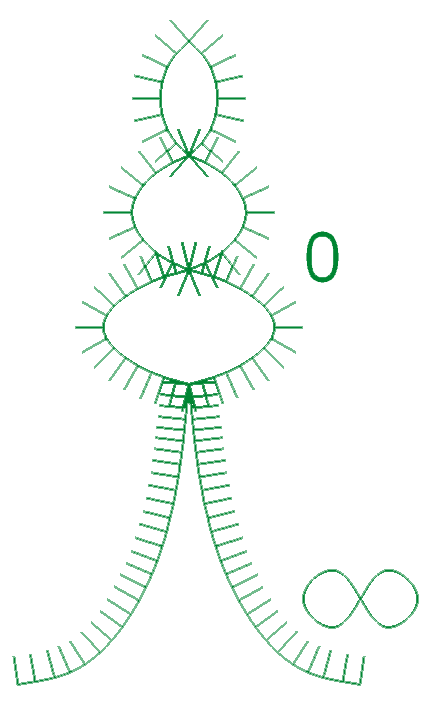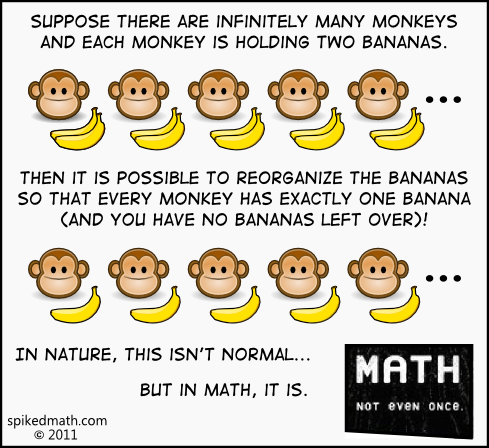Infinite Number Theorem

How AllA's Grand Unified Theory Connects to Define the Supreme Matrix Through the Infinite Number System According to Quantum Dynamics Theory!In the infinite number system numbers have values which vary across an infinite spectrum that both extends to infinitely greater infinities.... and extends to infinitely smaller 0s.... As such The Supreme Matrix encompasses all possible configurations of universes as long as the infinite number system can theoretically work. Which it does because all theories work as long as they remain logically consistent regardless of the postulates or the ramifications. The only postulate that the infinite number system violates is Sir Isaac Newton's Limit Notation which should forever be stricken from any mathematical theory unless in relevance as a historical fact. The mechanics behind all light can be described by the on particle interactions of AllA's Grand Unified Theory. Due to the infinite complexity of Quantum Dynamics Theory the variables tree into an infinite assortment of factors which contribute to the definition of universes described in AllA's Grand Unified Theory. Eventually quantum particles will diverge in complexity to phons of a complete spectrum of electromagnetic radiation whose frequencies range along the entire infinite number system irrational number line. Infinite universes will be generated in this one and infinite in those .. finite levels deep to complete the journey of all quantum beings. Due to the nature of the Infinitely Deep Universe Generator Fractal the spiritual factors of the souls in the universe most notably the soul of all life at nirvana are applicable in the definition of this universe and this is caused by the more complicated dynamics of Quantum Dynamics Theory in the more complex variables involved in those situations.

All Flaws in Quantum Mechanics stem from infinite values or 0 values of variables which cause failures at such values like electron density at proton cores, the density of the center of a black hole, and what happens when waves like light or quanti fan out to nothing infinitely far away and can easily be fixed by calculating all other values to figure out the probability of an electron being at a proton core by experiment for example thus eliminating all problems in Quantum Mechanics described in Supreme Matrix Theory!

Otherwise There Must Be A Fundamental Problem With Science/Math When You-But In The Infinite Number System The Monkeys Just Each Ate A Banana As Would Be Expected Leaving Infinite Banana Peals Behind And In This Case The Number of Monkeys Would Be The Same As The Number Of Banana Peals Left Behind And The Same As The Remaining Bananas. Thus The Number of Bananas There Before The Monkeys Each Ate 1 Would Be 2 Times The Number of Monkeys. Rather Than Ignoring That Unreal Numbers Have Definitive Values Even Though They Are Not Known Yet Try Knowing Them Better Without Promising Some New Fundamental Thing That Would Fix A Problem That Isn't There Without Having An Inkling Clue About What These Changes Are Or Would Be And Instead Fix The Monkey Business With Some Hard Science Called Relativity Of Infinities!

Supreme Matrix Theory

Grand Unified Theory

HealPacks

Main Page